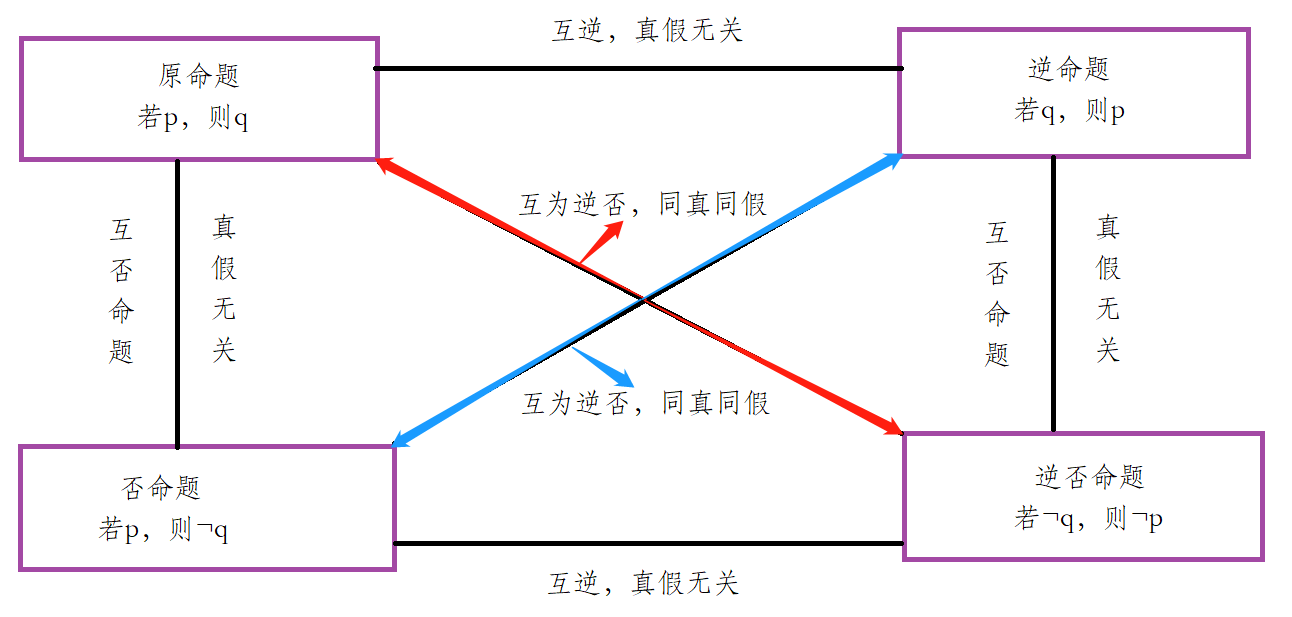# 元组关系演算（从集合的角度深入浅出）

## 一、定义

​ 元组关系演算中，以元组为单位，通过公式约束所要查找元组的条件，可以表示为: ${t\ |\ \psi(t)}$，使φ(t)为真的元组t的集合。其中: t为元组变量，即查询目的，φ为元组演算的谓词公式，即查询的条件。

## 二、元组关系演算的谓词公式

$\psi(t)$ 可以通过原子公式、约束公式、自由变量、运算符构成。

### （Ⅰ）原子公式分为三类

①、$R(t)$$R$为关系名，表示$t$$R$中的元组；

②、$t[\ i\ ]\quad\theta\quad u[\ j\ ]$：元组$t$的第$i$个分量与元组$u$的第$j$个分量进行比较运算，运算符号用$\theta$来表示。比如$t[\ 2\ ]\quad\lt\quad u[\ 3\ ]$

③、$t[\ i\ ]\quad\theta\quad C$：元组的第$i$个分量与常量$C$进行比较运算，运算符号用$\theta$来表示。比如$t[\ 2\ ]\quad\lt\quad 5$

### （Ⅲ）为此公式$\psi(t)$的递归定义

①、原子公式是公式；

②、通过吸取连接词、合取连接词所构成的复合公式也是公式。
$\psi_1(t_1)$$\psi_2(t_2)$是公式，则¬$\psi_1(t_1)$$\psi_1(t_1)\ \bigwedge\ \psi_2(t_2)$$\psi_1(t_1)\ \bigvee\ \psi_2(t_2)$也是公式；

③、利用全称量词和存在量词构成的公式也是$\psi(t)$的一种表现形式 。
$【(\exists\ t)\ \psi(t)】$$【(\forall\ t)\ \psi(t)】$也是公式;

④、有限次利用上述规则得到的式子都是公式；

## 三、公式运算符及其优先级

（Ⅰ）算术比较符: <, >, ≥, ≤, ≠, =；

（Ⅱ）全称量词$\forall$和存在量词$\exists$

（Ⅲ）逻辑运算符: $\bigwedge$, $\bigvee$, ¬, $\longrightarrow$

（Ⅳ）优先级: 算术比较符 > 全称量词$\forall$和存在量词$\exists$ > 逻辑运算符；

## 四、元组关系演算与关系代数

​ 在元组关系演算中，可以不用考虑为解决表的自然连接中产生数据冗余而进行投影属性列。
（注意：最好还是考虑，但是为了简化步骤就没有考虑）。

### （Ⅰ）传统关系代数 到 元组关系演算

①、交操作：$\{t | R(t)\ \bigwedge\ S(t)\}$;

②、并操作：$\{t | R(t)\ \bigvee\ S(t)\}$;

③、差操作：$\{t | R(t)\ \bigwedge\ ¬S(t)\}$;

④、广义笛卡尔积操作：$\{\ t(m+n)\ |\ (∃u)(∃v)\ （R(u) Λ S(v)）\ \bigwedge\ （t[\ i\ ]=u[\ j\ ]\ (i=1, 2, ..., m）\ \bigwedge\ t[\ i\ ]=v[\ k\ ])\ \}$

### （Ⅱ）专门关系代数 到 元组关系演算

（1） 选择操作：$\{t\ |\ R(t)\ \bigwedge\ F\}$，其中$F$是指查询条件。选取操作只是在原有的表上，将满足特定性质的元组提取出来，并没有形成新的表。

（2）投影操作: $\{t(n)\ |\ (∃u)\ (R(u)\ \bigwedge\ t[\ i\ ]\ =\ u[\ j\ ])\}$，其中$(i=1, 2, ..., n, j∈[1, count_A(R)])$

补充

## 五、浅析打开全称量词∀和存在量词∃的方式

（Ⅰ）存在量词∃：当需求中含有“存在一个”、“至少一个”、“有一个”等词的时候。就像上述的投影操作一样：有且仅有一个$R$中的$u$元组，满足$t[\ i\ ]\ =\ u[\ j\ ]$

（Ⅱ）全称量词∀：当需求中含有“全部”、“所有”等词，并且将“全部”一词去掉有后改变原句句意的时候。eg：

①、“在进行操作的过程中选择某一集合中全部元素”的元素集合时，即此时的“全部”并不是默认值，默认值为“至少一个 / 存在”，比如说某个要求为：“查询选修了全部课程的学生”，此时将“全部”一词去掉后变为“查询选修了课程的学生”，不难发现，前一个是“选修了全部课程”，后一个是“只要是选修了课程，即至少选修了一门课程”，将两者比较，两句话的句意完全不同。

②、在经过一系列操作后所得到的集合中选择全部元组时，即此时的“全部”为默认值：对于“查询选修了‘刘伟’老师的课程的全部学生”，将“全部”一词去掉后变为“查询选修了‘刘伟’老师的课程的学生”，这并没有$len$何差别，所以自然就“不配”使用“全称量词∀”了。

补充

## 六、命题的否定 VS 否命题

### （Ⅱ）否命题: 若$¬p$，则$¬q$。（$PASS$）### （Ⅲ）全称量词∀和存在量词∃的否定形式

（1）全称量词∀的否定形式

①、更换量词: 将全称量词∀换成存在量词∃；
②、将结论否定；

（2）存在量词∃的否定形式

①、更换量词: 将存在量词∃换成全称量词∀；
②、将结论否定；

## 七、总结，深入全称量词∀和存在量词∃的打开方式

（1）“相离” 与“相交”∪“包含”：互为对立事件，即“全部都没” $VS$ “至少有一”；
（2）“包含” 与“相交”∪“相离”：互为对立事件，即“全部都有” $VS$ “至少没一”；

（1）当某个事件被表述为两个集合“相离”或者“包含”的时候 ：使用“全称量词∀”来表示“全部都没”或者“全部都有”；
（2）当某个事件被表述为两个集合“相交”的时候：使用“存在量词∃”来表达“至少有一”或者“至少没一”;

（1）相离“没有选修过‘李力’老师教授的课程的同学”这个问题就可以被表述为：$A_i ∩ B = \phi$，其中$A_i$ = {第$i$个学生的“学生-选课信息”的课程编号集合}，$B$ = {‘李力’老师教授的课程的课程编号集合}；

（2）相交“选修过‘李力’老师教授的课程的同学”这个问题就可以被表述为：$A_i ∩ B ≠ \phi$，其中$A_i$ = {第$i$个学生的“学生-选课信息”的课程编号集合}，$B$ = {‘李力’老师教授的课程的课程编号集合}；

（3）包含“选修了‘李力’老师教授的全部课程的同学”这个问题就可以被表述为：$A_i$$B$，其中$A_i$ = {第$i$个学生的“学生-选课信息”的课程编号集合}，B = {‘李力’老师教授的课程的课程编号集合}；

（1）当考虑的问题是“全部都有”时则可以从正面入手，在关系代数中利用除法，在元组演算中利用“全称量词∀”

（2）当考虑的问题是“至少没有一个”时则可以从反面入手，在关系代数中利用除法 + 减法，在元组演算则从正面入手，直接利用“存在量词∃”，译作“存在一个没有满足……”；

（3）当考虑的问题是“至少存在一个”时则可以从正面入手，在关系代数中利用自然连接，在元组演算中利用“存在量词∃”，译作“存在一个满足……”；

（4）当考虑的问题是“全部都无”时则可以从反面入手，在关系代数中利用自然连接 + 减法，在元组演算中利用“全称量词∀”；

## 八、蕴含式（包括existsS相关子查询的分析）

posted @ 2020-07-05 23:29  BN2U  阅读(519)  评论(0编辑  收藏